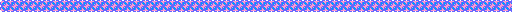R&D of Mesoscale Particle Simulation Code

For high-temperature plasma simulations, one needs
several types of codes to handle
macroscopic (large scale)
and
microscopic (small scale) phenomena. The mesoscale
particle code is aimed to deal with
plasma kinetic
phenomenon
both with medium time and space scales.

Why Mesoscale Particle Code ?

Since we have to keep track of particles motion along their orbits,
we start with a particle code.　However, a plasma has an eigenmode
called
"plasma" (Langmuir) oscillations, whose time and space scales
are of the plasma frequency and the Debye length, respectively, which
need be resolved (even if one is not interested in them) for stable
numerical simulations. 　Great deal of efforts of removing these small-
scale oscillations have been taken
in the United States in early 1980s,
especially in Los Alamos and Livermore National Laboratories.

The former group makes use of fluid equations and
the backward
time discretization technique
to filter out plasma oscillations, where
particle contributions are counted as the first and second moment
source terms to the equations.　The latter group devised high accuracy
numerical lowpass filter to plug in to a particle code.　The pros and
cons of these methods are numerical stability and substantial
computation load for the former, and simplicity of the algorithm but
numerical instability for the latter.

What is New with My Algorithm ?

Since I started developing a mesoscale particle code, I could adopt
numerically stable
backward time discretization technique. 　My
invention is to represent all unknown (future) field quantities in the
equations by the already known quantities with aid of the Maxwell and
Newton equations of motion.
This rendered use of minimum a priori
assumptions in equations, numerical stability in general, and less
computational load than with the Los Alamos code.

The derived field equations to be solved are
fully implicit ones in
which unknown quantities appear on the leftside of the equations. The
size of the unknowns are three times the number of space grids.　For
spatial dimensions less or equal to two, the solution converges fairly
well.

However,
for three dimensions in space, the number of the
unknowns amounts to 300,000 even if there are only 50 grids along one
axis of the space, which posed numerical difficulty in solving such giant
the bi-conjugate
which is aimed to solve giant nonsymmetric matrix
equations,
that the 3D mesoscale particle code became available for
realistic plasma simulations.

The algorithm and accuracy proof/demonstrations of the mesoscale
particle code that I developed from nearly a scratch (called HIDEN)
are shown in the following papers.　The second paper describes three
dimensional cases with numerical techniques required in such
dimensions.

References:
Physics of High-Temperature Plasmas (in Japanese)
(Parity Physics Course, Maruzen; 1991, 1997)

Algorithm and proof of mesoscale particle code
J.Comput.Phys., 79, 209 (1988).

３D Mesoscale particle code
J.Comput.Phys.,　107, 124 (1993).

Research Purpose and Results 　　Home

Magnetic Reconnection